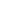## Classical mechanicsNewton's laws of motion are three physical laws that form the basis for classical mechanics. They describe the relationship between the forces acting on a body and its motion due to those forces. They have been expressed in several different ways over nearly three centuries, and can be summarized as follows:

1.First law: If an object experiences no net force, then its velocity is constant: the object is either at rest (if its velocity is zero), or it moves in a straight line with constant speed (if its velocity is nonzero).
2.Second law: The acceleration a of a body is parallel and directly proportional to the net force F acting on the body, is in the direction of the net force, and is inversely proportional to the mass m of the body, i.e., F = ma.
3.Third law: When a first body exerts a force F1 on a second body, the second body simultaneously exerts a force F2 = −F1 on the first body. This means that F1 and F2 are equal in magnitude and opposite in direction.
The three laws of motion were first compiled by Sir Isaac Newton in his work Philosophiæ Naturalis Principia Mathematica, first published in 1687. Newton used them to explain and investigate the motion of many physical objects and systems. For example, in the third volume of the text, Newton showed that these laws of motion, combined with his law of universal gravitation, explained Kepler's laws of planetary motion.
Theory Explanation:
Newton's laws are applied to bodies (objects) which are considered or idealized as a particle, in the sense that the extent of the body is neglected in the evaluation of its motion, i.e., the object is small compared to the distances involved in the analysis, or the deformation and rotation of the body is of no importance in the analysis. Therefore, a planet can be idealized as a particle for analysis of its orbital motion around a star.
In their original form, Newton's laws of motion are not adequate to characterize the motion of rigid bodies and deformable bodies. Leonard Euler in 1750 introduced a generalization of Newton's laws of motion for rigid bodies called the Euler's laws of motion, later applied as well for deformable bodies assumed as a continuum. If a body is represented as an assemblage of discrete particles, each governed by Newton’s laws of motion, then Euler’s laws can be derived from Newton’s laws. Euler’s laws can, however, be taken as axioms describing the laws of motion for extended bodies, independently of any particle structure.
Newton's laws hold only with respect to a certain set of frames of reference called Newtonian or inertial reference frames. Some authors interpret the first law as defining what an inertial reference frame is; from this point of view, the second law only holds when the observation is made from an inertial reference frame, and therefore the first law cannot be proved as a special case of the second. Other authors do treat the first law as a corollary of the second. The explicit concept of an inertial frame of reference was not developed until long after Newton's death.
In the given interpretation mass, acceleration, momentum, and (most importantly) force are assumed to be externally defined quantities. This is the most common, but not the only interpretation: one can consider the laws to be a definition of these quantities.
Newtonian mechanics has been superseded by special relativity, but it is still useful as an approximation when the speeds involved are much slower than the speed of light.

Labels:
•    Type→ Basic Science
•    Theorist→ Isaac Newton
•    Date →1687

### Online visitor

We have 101 guests and no members online